Test: Sequential Logic Circuits- 1

# Test: Sequential Logic Circuits- 1

Test Description

## 10 Questions MCQ Test GATE Electrical Engineering (EE) 2023 Mock Test Series | Test: Sequential Logic Circuits- 1

Test: Sequential Logic Circuits- 1 for Electronics and Communication Engineering (ECE) 2023 is part of GATE Electrical Engineering (EE) 2023 Mock Test Series preparation. The Test: Sequential Logic Circuits- 1 questions and answers have been prepared according to the Electronics and Communication Engineering (ECE) exam syllabus.The Test: Sequential Logic Circuits- 1 MCQs are made for Electronics and Communication Engineering (ECE) 2023 Exam. Find important definitions, questions, notes, meanings, examples, exercises, MCQs and online tests for Test: Sequential Logic Circuits- 1 below.
Solutions of Test: Sequential Logic Circuits- 1 questions in English are available as part of our GATE Electrical Engineering (EE) 2023 Mock Test Series for Electronics and Communication Engineering (ECE) & Test: Sequential Logic Circuits- 1 solutions in Hindi for GATE Electrical Engineering (EE) 2023 Mock Test Series course. Download more important topics, notes, lectures and mock test series for Electronics and Communication Engineering (ECE) Exam by signing up for free. Attempt Test: Sequential Logic Circuits- 1 | 10 questions in 30 minutes | Mock test for Electronics and Communication Engineering (ECE) preparation | Free important questions MCQ to study GATE Electrical Engineering (EE) 2023 Mock Test Series for Electronics and Communication Engineering (ECE) Exam | Download free PDF with solutions
 1 Crore+ students have signed up on EduRev. Have you?
Test: Sequential Logic Circuits- 1 - Question 1

### For which of the following flip-flops, the output is clearly defined for all combinations of two inputs?

Detailed Solution for Test: Sequential Logic Circuits- 1 - Question 1

For J-K flip-flop, the output is clearly defined for all combinations of two inputs. For S-R flip-flop output is not defined when S = R = 1. A D flip-flop has only one input.

Test: Sequential Logic Circuits- 1 - Question 2

### What J-K input condition will always set ‘Q+ upon the occurrence of the active clock transition ?

Detailed Solution for Test: Sequential Logic Circuits- 1 - Question 2

Truth table for J-K flip-flop is shown below.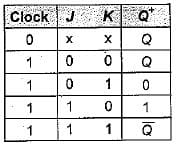When J = 1 and K = 0, output (Q+) is always set upon the occurrence of the active clock transition.

Test: Sequential Logic Circuits- 1 - Question 3

### In a J-K flip-flip, toggle means

Detailed Solution for Test: Sequential Logic Circuits- 1 - Question 3

In a J-K flip-flop, toggle means change the output to the opposite state. A J-K flip-flop toggles when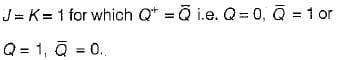Test: Sequential Logic Circuits- 1 - Question 4

The output of S-R flip-flop when S = 1, R = 0 is

Detailed Solution for Test: Sequential Logic Circuits- 1 - Question 4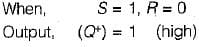Test: Sequential Logic Circuits- 1 - Question 5

An eight stage ripple counter uses a flip-flop with propagation delay of 75 nano-seconds. The pulse width of the strobe is 50 nano-seconds. The frequency of the input signal which can be used for proper operation of the counter is approximately equal to

Detailed Solution for Test: Sequential Logic Circuits- 1 - Question 5

Maximum time taken for all flip-flops to stabilize is (75 ns x 8) + 50 ns = 650 ns.
Frequency of operation must be less than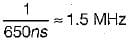Thus, option (d) is correct.

Test: Sequential Logic Circuits- 1 - Question 6

The output of a J-K flip-flop with asynchronous preset and clear inputs if ‘1 ’. The output can be changed to ‘0’ with which one of the following conditions?

Detailed Solution for Test: Sequential Logic Circuits- 1 - Question 6

When J = 1, K = 1 and the clock, next state will be complement of the present state. Thus,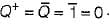Test: Sequential Logic Circuits- 1 - Question 7

A J-K flip-flop can be implemented using D flip- flop connected such that

Test: Sequential Logic Circuits- 1 - Question 8

Assertion (A): In general, asynchronous circuits are considerably faster than synchronous circuits.
Reason (R): In an asynchronous circuit, events can occur after one event is completed and there is no need to wait for a clock pulse.

Test: Sequential Logic Circuits- 1 - Question 9

Consider the following statements:
1. Sequential circuits are always faster than combination circuits.
2. In an asynchronous circuit there is no problem of stability.
3. The logic circuits whose outputs at any instant of time depend only on the input signals present at that time are known as combinational circuits.
4. In a combinational circuit, for a change in the input, the output appears immediately.
5. In a sequential circuits, the output signals are fed back to the input side.

Which of the statement given above are correct?

Detailed Solution for Test: Sequential Logic Circuits- 1 - Question 9

• Combinational circuits are often faster than sequential circuits since the combinations circuits do not require memory whereas the sequential circuits need memory devices to perform their operations in sequence. Hence statement - 1 is not correct.
• in an asynchronous circuit, events are allowed to occur without any synchronisation In such a case, the system become: unstable which results in difficulties. Hence statement-2 is not correct.
• Statement-3 is correct which is the definitioi of a combinational circuit.
• in a combinational circuit, for a change if the input, the output appears immediately except for the propagation delay througt circuit gates. Thus, statement-4 is no correct.
• In a sequential circuit, an output signal is e function of the present input signals and e sequence of the past input signals i.e. the past output signals since the output signals are fed back to the input side. Hence, statement-5 is correct.
Thus, statements 3 and 5 are only correct.

Test: Sequential Logic Circuits- 1 - Question 10

Assertion (A): A latch is a memory device with the capability of storing one binary digit of information.
Reason (R): A basic latch is made up of cross coupled inverters.

Detailed Solution for Test: Sequential Logic Circuits- 1 - Question 10

A latch is memory device with the capability of storing one binary digit of information because the latch output will remain set/reset until the trigger pulse is given to change the state.
A basic latch is made up of cross coupled inverters as shown below.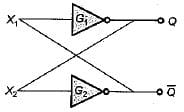Thus, both assertion and reason are true but reason is not the correct explanation of assertion.

## GATE Electrical Engineering (EE) 2023 Mock Test Series

22 docs|274 tests
Information about Test: Sequential Logic Circuits- 1 Page
In this test you can find the Exam questions for Test: Sequential Logic Circuits- 1 solved & explained in the simplest way possible. Besides giving Questions and answers for Test: Sequential Logic Circuits- 1, EduRev gives you an ample number of Online tests for practice

## GATE Electrical Engineering (EE) 2023 Mock Test Series

22 docs|274 tests(Scan QR code)